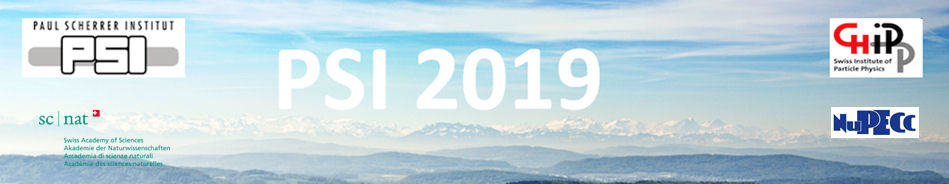#Physics of fundamental Symmetries and Interactions - PSI2019

Oct 20 – 25, 2019
PSI
Europe/Zurich timezone

## The $a$SPECT experiment - Overview of systematic uncertainties

Oct 22, 2019, 5:09 PM
1m
WHGA/001 - Auditorium (PSI)

Poster

### Speaker

Dr Christian Schmidt (Johannes Gutenberg-Universität Mainz)

### Description

The $a$SPECT experiment is a retardation spectrometer built to measure the proton energy spectrum in free neutron $\beta$-decay. From the shape of the spectrum, the $\beta$-$\overline{\nu}_e$ angular correlation coefficient $a$ can be derived and thus $\lambda(a)$, the ratio of the weak axial-vector $(A)$ to vector $(V)$ coupling constant $\lambda=g_A/g_V$.

In 2013, $a$SPECT had a successful beam time at the Institut Laue-Langevin, Grenoble (France). Different parameter settings of the spectrometer helped to trace instrumental systematics. Supportive follow-up measurements were conducted, e.g. to determine the spatial and temporal work function fluctuations of the electrodes, which is a source of one of the main systematic uncertainties. These measurements were used as input for electromagnetic field computations and particle tracking simulations for a correction of systematics. The data of the runs in the individual configurations were combined and analysed using a multi-dimensional fit with $a$ as free fit parameter.

From this beam time we obtain $a=-0.10430(84)$ $\left[1\right]$ which is the most precise measurement of the neutron $a$ coefficient to date (PDG2018 value $a=0.1059(28)$). The ratio of axial-vector to vector coupling constant is derived giving $|\lambda(a)|=1.2677(28)$. The sources of systematic uncertainties are considered and included in the results.

This poster gives an overview of the systematic uncertainties. Special focus is given on the systematics that could only be quantified by sophisticated electromagnetic field and particle tracking simulations as part of my Ph.D. thesis $\left[2\right]$.

$\left[ 1\right]$ M. Beck et al., arXiv:1908.04785 [nucl-ex], 13 Aug 2019, submitted to Phys. Rev. C
$\left[2\right]$ C. Schmidt, Ph.D. thesis, Johannes Gutenberg-Universität Mainz, 2019.

### Primary author

Dr Christian Schmidt (Johannes Gutenberg-Universität Mainz)